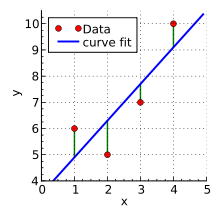@Pigmon 2017-10-20T06:19:03.000000Z 字数 559 阅读 160

# 最小二乘法线性回归

教案

### 维基百科上最小二乘法应用在线性回归上的例子$k\times 1 + b=6$
$k\times 2 + b=5$
$k\times 3 + b=7$
$k\times 4 + b=10$

$S(k,b) = [6-(k + b)]^2+[5-(2k + b)]^2+[7-(3k + b)]^2+[10-(4k + b)]^2$

$\frac{\partial S}{\partial b}=8b+20k-56=0$
$\frac{\partial S}{\partial k}=20b+60k-154=0$

$b=3.5,k=1.4$• 私有
• 公开
• 删除